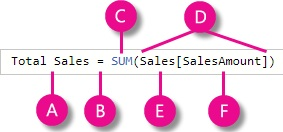# Understand DAX in Power BI

#### What is a DAX ?

Data Analysis Expressions (DAX) is both a query and functional language.
The primary objective of DAX is to help organize, analyze, understand, and enhance data for analytics and reporting.

#### Why to use DAX in Power BI ?

DAX helps in creating new information from existing information or data.
The existing information can be at detail level, using DAX we can create information such as aggregated, summarized and so on.

#### How to implement DAX in Power BI ?

Before implementing DAX in Power BI the following things need to be kept in mind:

• DAX works on column values of a Table.
• DAX cannot modify or insert data, that means source data is SAFE.
• We can create calculated column and measures with DAX but we cannot calculate rows using DAX.

Syntax of DAX :The above pic is formula of DAX and details of syntax is shown below

A : is the measure name as 'Total Sales'.

B : The equals sign operator (=) indicates the beginning of the formula. When calculated, it will return a result.

C : The DAX function 'SUM' is used to add all the numbers in the Sales[SalesAmount] column.

D : Parenthesis () surround an expression containing one or more arguments. All functions require at least one argument.
An argument passes a value to a function.

E :is the table name as 'Sales' which is been referred.

F : The referenced column [SalesAmount] in the Sales table.
With this argument, the SUM function knows on which column to aggregate a SUM.

Some of the commonly used DAX Functions :

• SUM, MIN, MAX, AVG
• IF, AND, OR, SWITCH
• UNION, INTERSECT, NATURALINNERJOIN, NATURALLEFTOUTERJOIN, ISEMPTY
• VALUES, ALL, CALCULATE
• MEDIAN, COUNTROWS, DATEDIFF, VAR(Variables)

Order only MSBI self-study learning video materials which are available with customized package costing 999 INR/15\$. Click this link here to see details and order it.